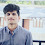# Class X NCERT (CBSE) Guide - Mathematics

CBSE Guide NCERT Solutions >> Class 10 NCERT Answers

## CBSE Class 10, Mathematics : NCERT Solutions

Welcome to !
• Chapter wise NCERT solutions of CBSE Class X Mathematics textbook exercises.
• A free CBSE Guess and Guide with additional practice questions important for CBSE Board Exam

### 10th Maths NCERT Solutions - Class X CBSE Mathematics Guide

NCERT Mathematics Textbook
Chapter Exercise / Lesson
NCERT Math Solutions

#### REAL NUMBERS

Exercise 1.1 (Page 7)
Exercise 1.2 (Page 11)
Exercise 1.3 (Page 14)
Exercise 1.4 (Page 17, 18)

#### POLYNOMIALS

Exercise 2.1 (Page 28)
Exercise 2.2 (Page 33)
Exercise 2.3 (Page 36)
Exercise 2.4 (Page 36, 37)

#### PAIR OF LINEAR EQUATIONS IN TWO VARIABLES

Exercise 3.1 (Page 44)
Exercise 3.2 (Page 49, 50)

Exercise 3.3 (Page 53, 54)

Exercise 3.4 (Page 56, 57)

Exercise 3.5 (Page 62, 63)

Exercise 3.6 (Page 67)

Exercise 3.7 (Page 68)

Exercise 4.1 (Page 73, 74)
Exercise 4.2 (Page 76)
Exercise 4.3 (Page 87, 88)

Exercise 4.4 (Page 91)

#### ARITHMETIC PROGRESSIONS

Exercise 5.1 (Page 99, 100)
Exercise 5.2 (Page 105, 106, 107)
Exercise 5.3 (Page 112, 113, 114)

Exercise 5.4 (Page 115)

#### TRIANGLES

Exercise 6.1 (Page 122)
Exercise 6.2 (Page 128, 129)
(India Study Solution)
Exercise 6.3 (Page 138, 139, 140, 141)

Exercise 6.4 (Page 143, 144)

Exercise 6.5 (Page 150, 151)

Exercise 6.6 (Page 152, 153)

#### COORDINATE GEOMETRY

Exercise 7.1 (Page 161, 162)

Exercise 7.2 (Page 167)

Exercise 7.3 (Page 170)
Exercise 7.4 (Page 171)

#### INTRODUCTION TO TRIGONOMETRY

Exercise 8.1 (Page 181 )
Exercise 8.2 (Page 187)

Exercise 8.3 (Page 189, 190)

Exercise 8.4 (Page 193, 194)

#### SOME APPLICATIONS OF TRIGONOMETRY

Exercise 9.1 (Page 203, 204, 205)

#### CIRCLES

Exercise 10.1 (Page 209 )

Exercise 10.2 (Page 213, 214)

#### CONSTRUCTIONS

Exercise 11.1 (Page 219, 220)

Exercise 11.2 (Page 221, 222)

#### AREAS RELATED TO CIRCLES

Exercise 12.1 (Page 225, 226)
Exercise 12.2 (Page 230, 231)

Exercise 12.3 (Page 234 - 238)

#### SURFACE AREAS AND VOLUMES

Exercise 13.1 (Page 244, 245)

Exercise 13.2 (Page 247, 248)

Exercise 13.3 (Page 251, 252)

Exercise 13.4 (Page 257)

Exercise 13.5 (Page 258)

#### STATISTICS

Exercise 14.1 (Page 270, 271, 272)

Exercise 14.2 (Page 275, 276)

Exercise 14.3 (Page 287 - 289)

Exercise 14.4 (Page 293)

#### PROBABILITY

Exercise 15.1 (Page 308 - 311)

Exercise 15.2 (Page 311, 312)

1.why only first lesson????????????

2.why we can't read trignometry and triangle as they are the toughest chapeter in whole book

3.how to read this it's not working

4.hello sir
pls do give me the answer of chapter linear equation and trgnomentry, triangle

5.worst site ever......thumbsdown